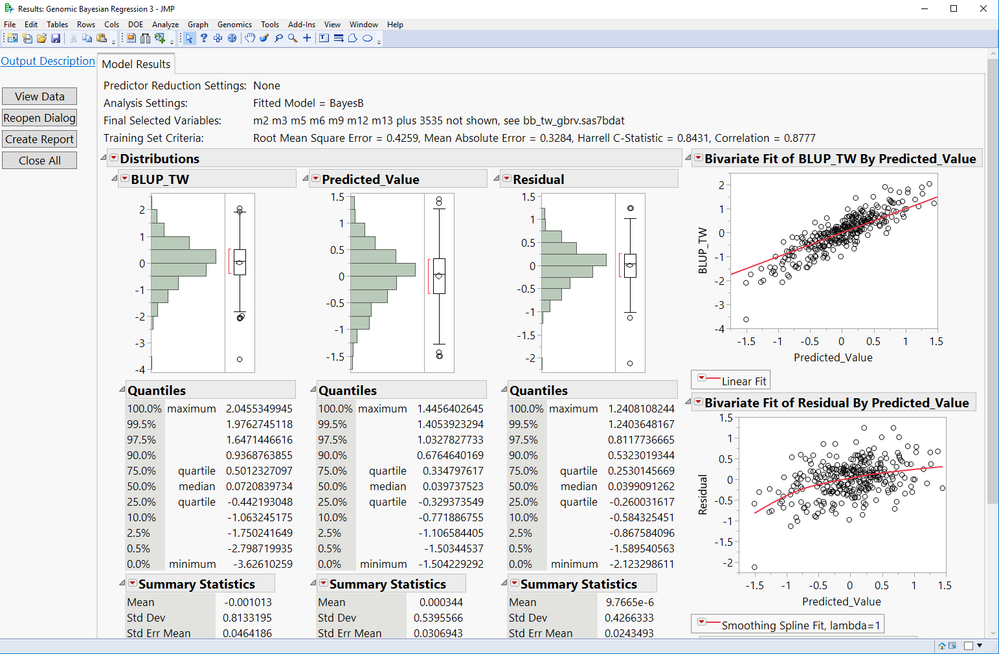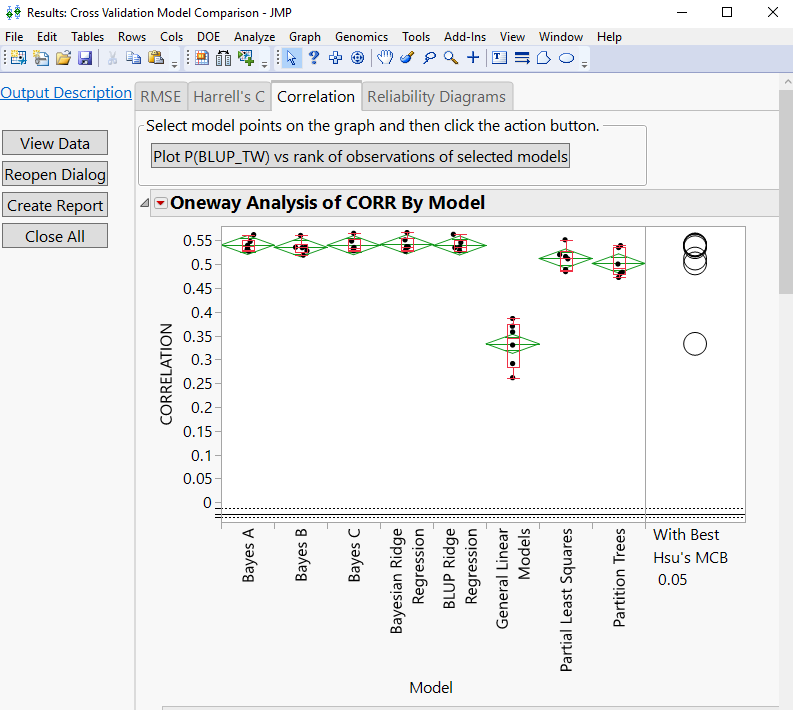Choose Language Hide Translation Bar

## Genomic Bayesian Methods Add-in for JMP® Genomics

The Genomic Bayesian Methods Add-in is a wrapper around the Bayesian Generalized linear Regression (BGLR; de los Campos and Rodriguez 2014, Genetics 198:483-495). Genetic marker effects can be estimated using Bayes A, Bayes B, Bayes C, Bayesian LASSO, or Bayesian Ridge Regression. The methods in this Add-In are fully integrated with the Cross Validation Model Comparison tool in JMP Genomics, allowing the comparison of accuracy of many distinct models. Additionally, the marker effects of the fitted models are saved in a score file that can be subsequently loaded in the Cross Evaluation and Progeny Selection breeding tools in JMP Genomics to predict breeding values of individuals, evaluate crosses, and simulate progenies in multiple generations of breeding.

Figure bellow shows the Bayes B method fitted to a barley data set. Notice the correlation between predicted and observed trait values is 0.8777.Figure bellow shows the average of correlation between predicted and observed trait values obtained by 3-fold cross validation ran for 6 iterations. Therefore, the correlation shown in the plot is the average taken over 18 runs for each tested model. While the average correlation for Bayes B is 0.5357 in the cross validation runs, the correlation for Bayes B in the entire data set without cross validation is 0.8777 (shown in the first figure). This example shows the importance of comparing models through cross validation to get more realistic measure of predictive accuracy.Article Labels
Article Tags
Contributors